### How to solve a Square-0

Learn how to solve a SQ-0 (aka Square-0), a shape mod of the original Square-1. This non-WCA puzzle is extremely fun and a great puzzle for those looking to get into Square-1 but may not want to jump straight in.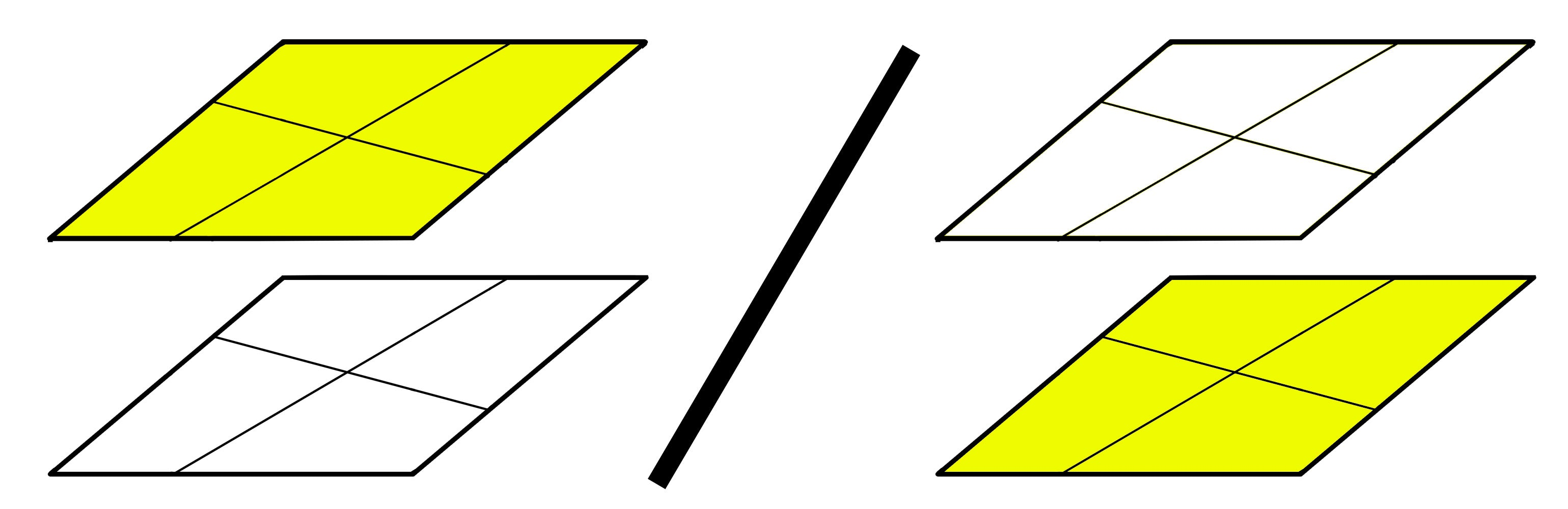### Top & Bottom Colour

Now, you may have white on the top or yellow on the top depending on your cube.
In this "How to solve a SQ-0" guide, we will be using yellow on the top. If you do have white on the top then you can follow along and use the same algorithm but your colours will be inverted (yellow on bottom and white on top).

### Step 1

In step 1 we will turn our Square-0 into a cube shape and orientate our corners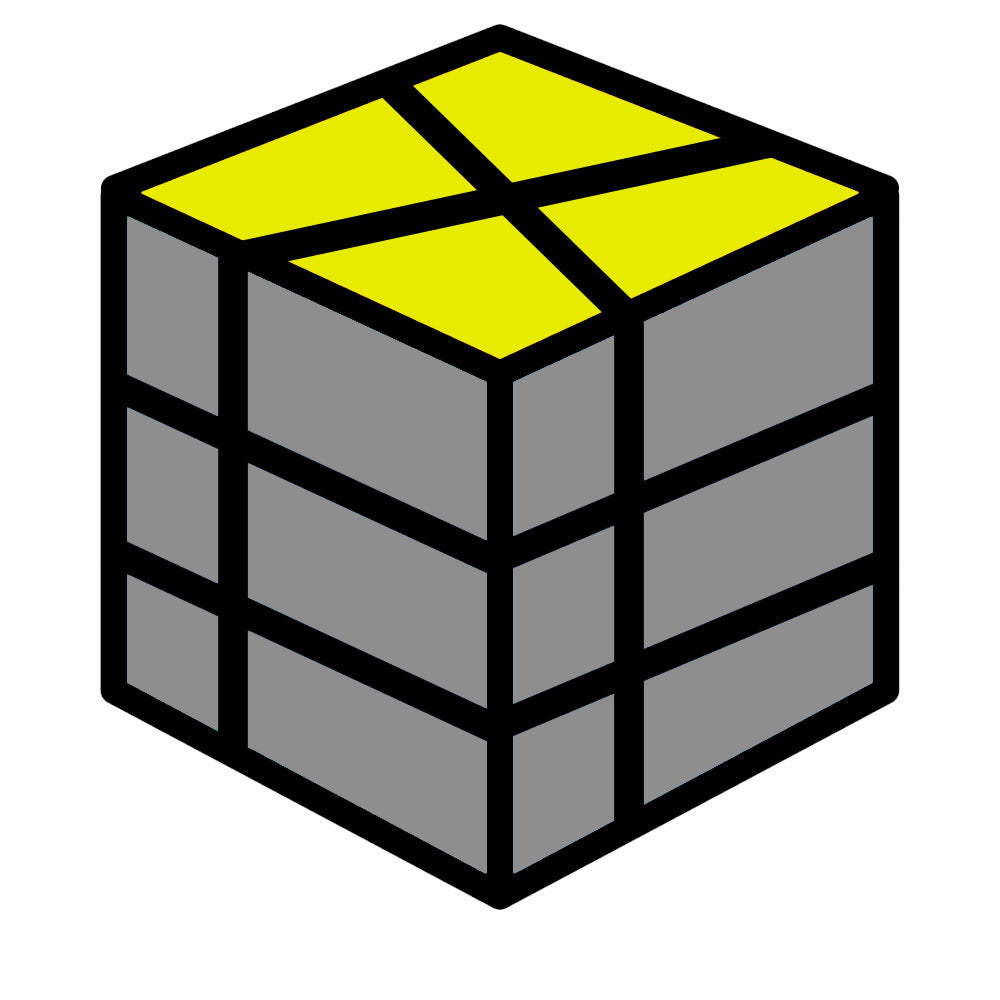### Step 2

In step 2 we are going to permute our corners on the top and bottom layer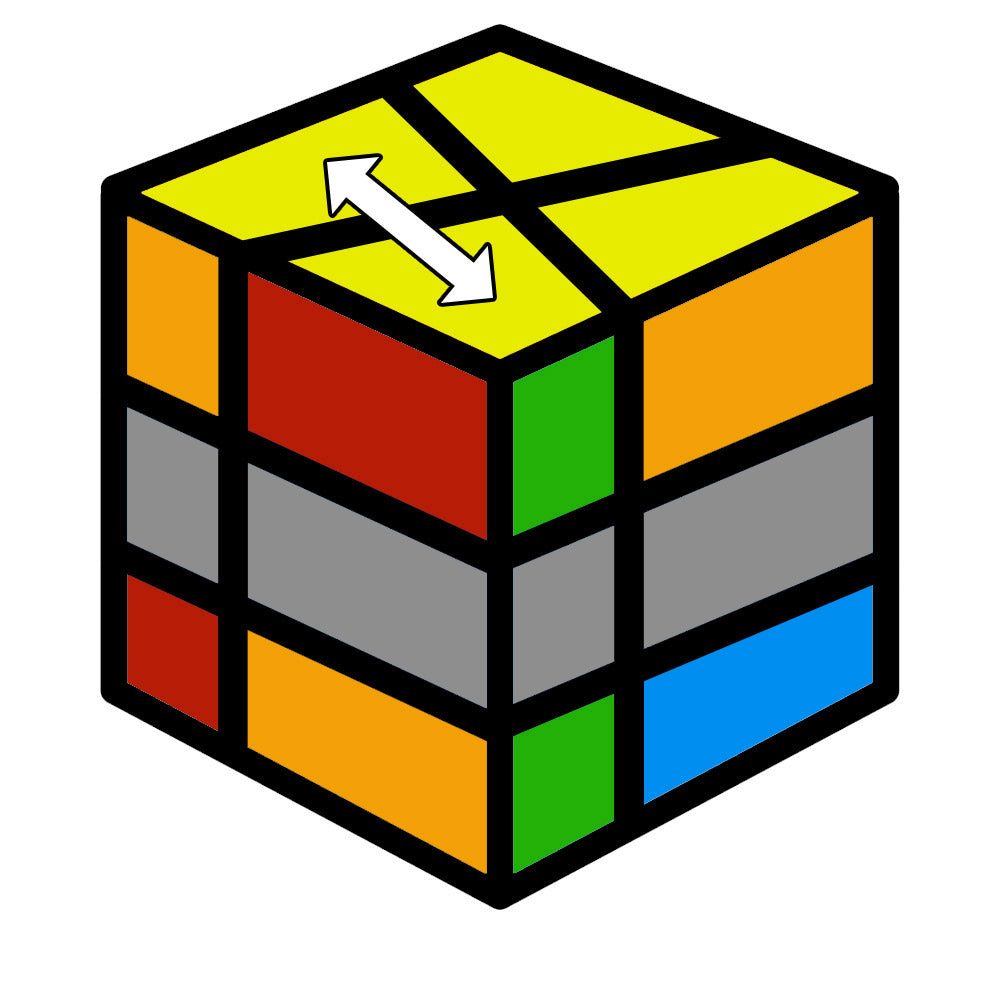### Step 3

Step 3 is optional - We will fix any issues relating to the center layer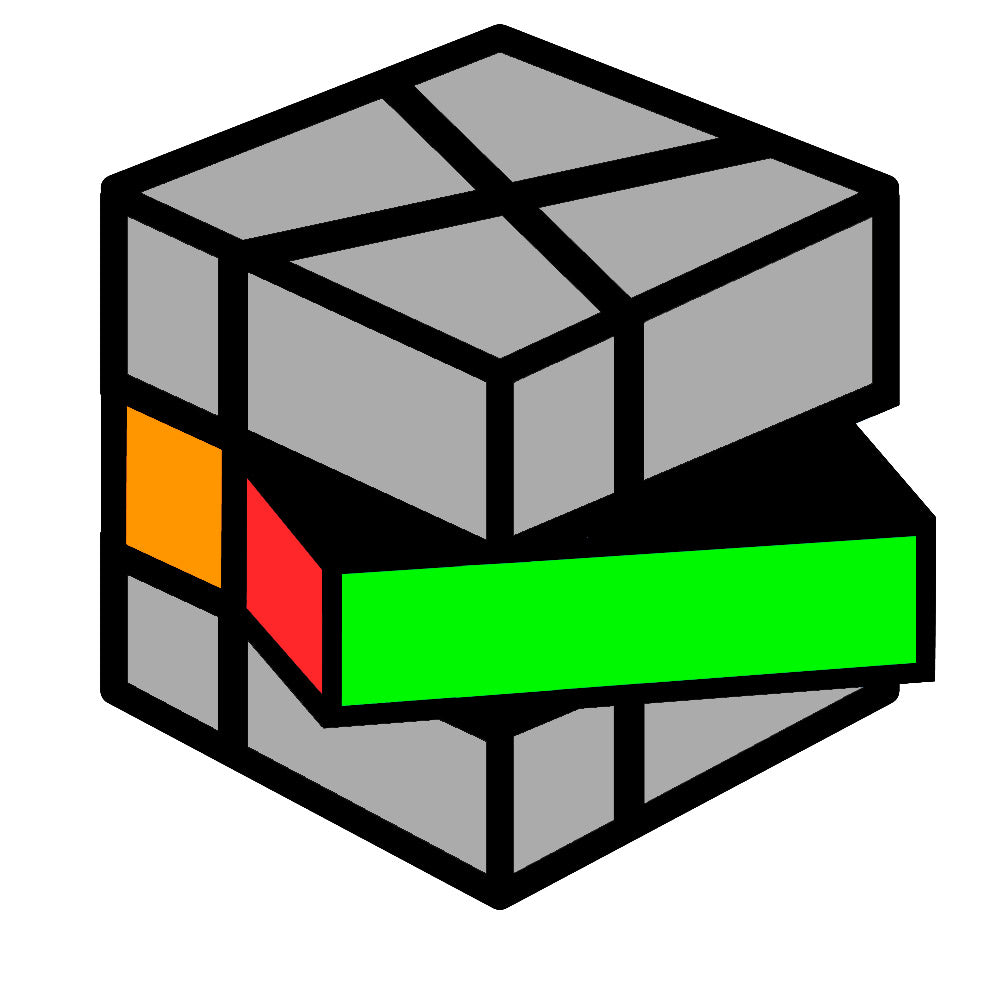# Step 1

Let's make a cube shape and orientate our corners. At the end of this step your middle slice may be incorrect, if so, don't worry and just carry on to step 2.

Note: The top image in each case below is the top layer of your SQ-0 and the bottom image is the bottom layer. You are matching the SHAPES and NOT the COLOURS.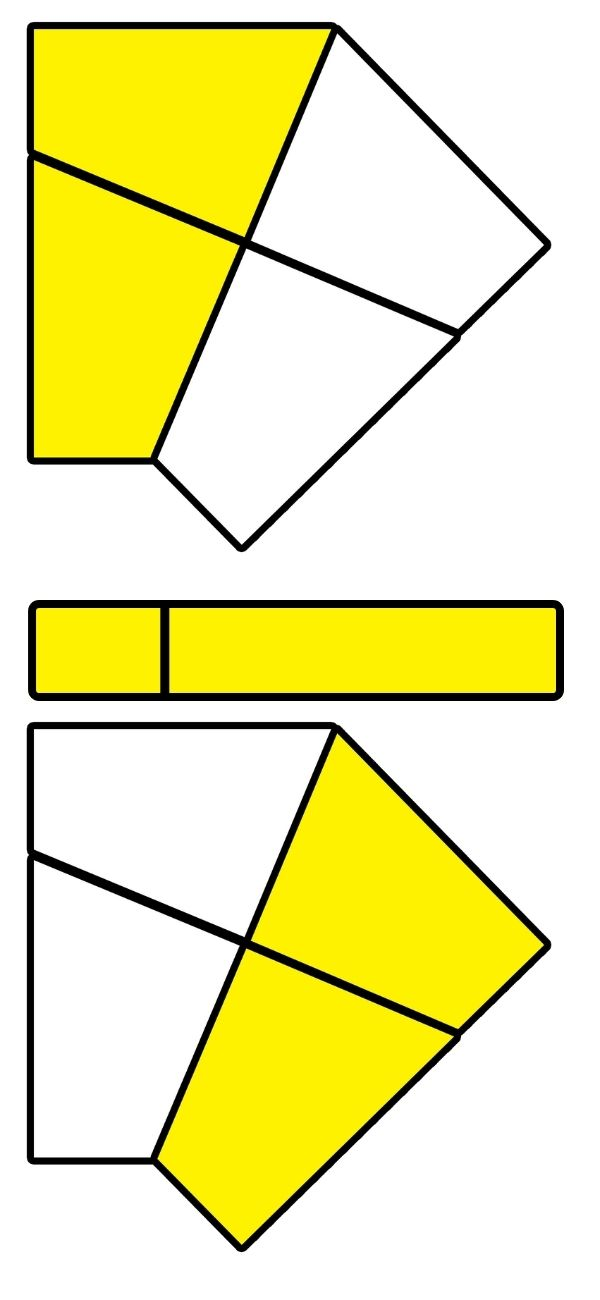### Case 1

/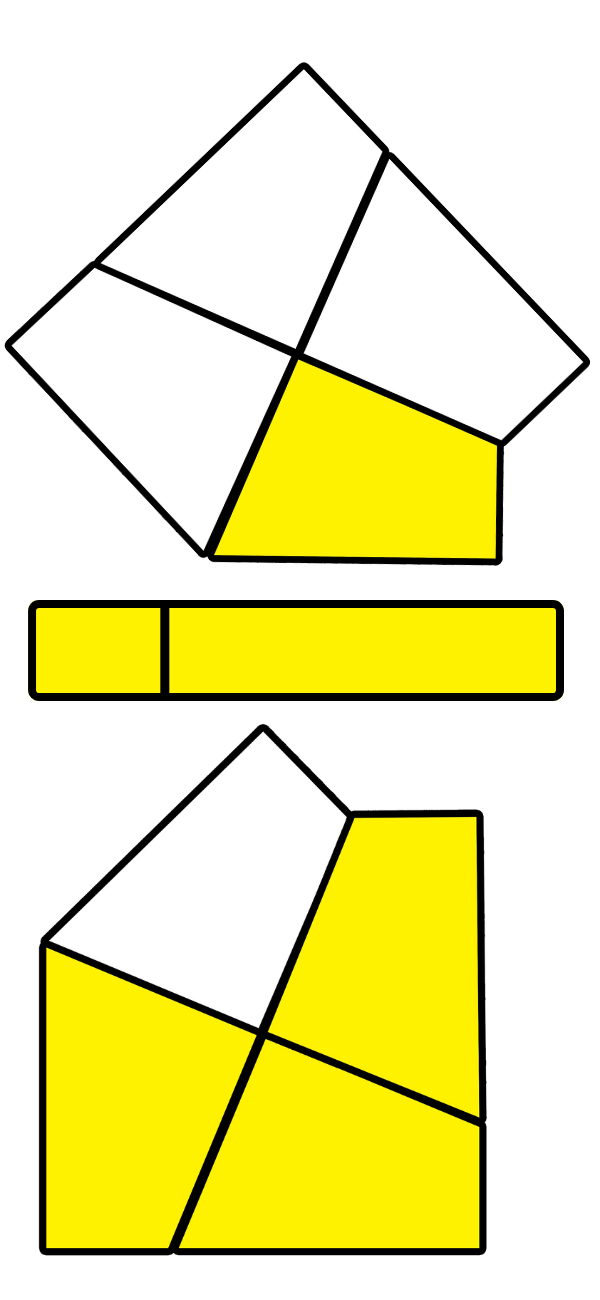### Case 2

/ (0,3) /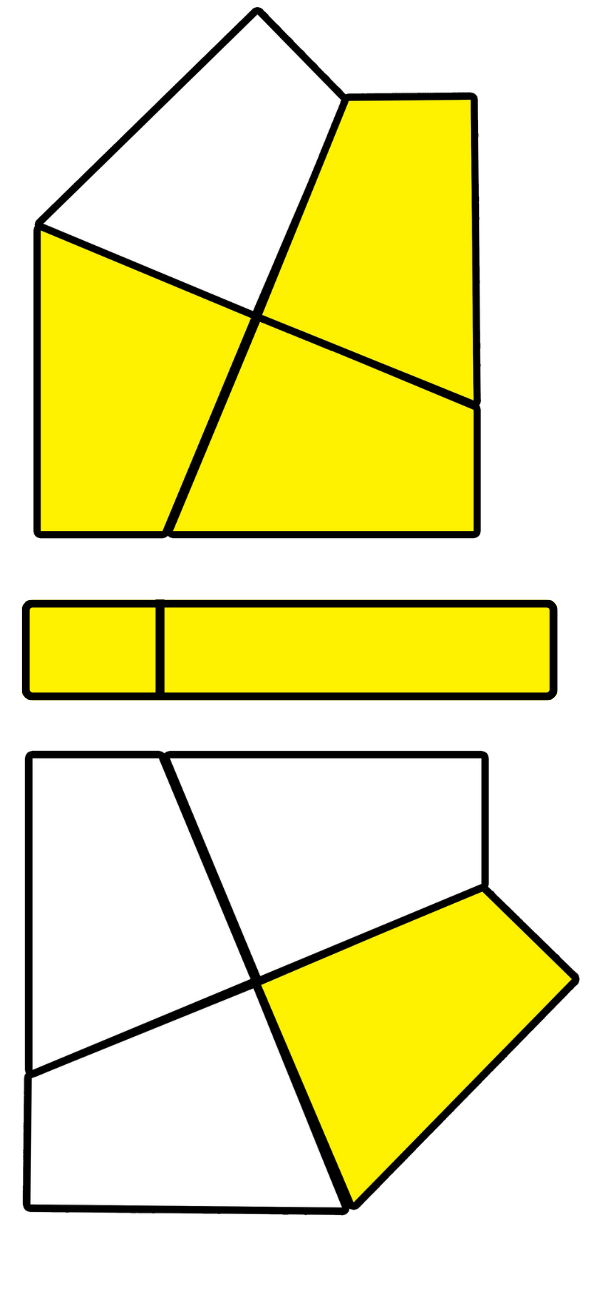### Case 3

/ (3,0) /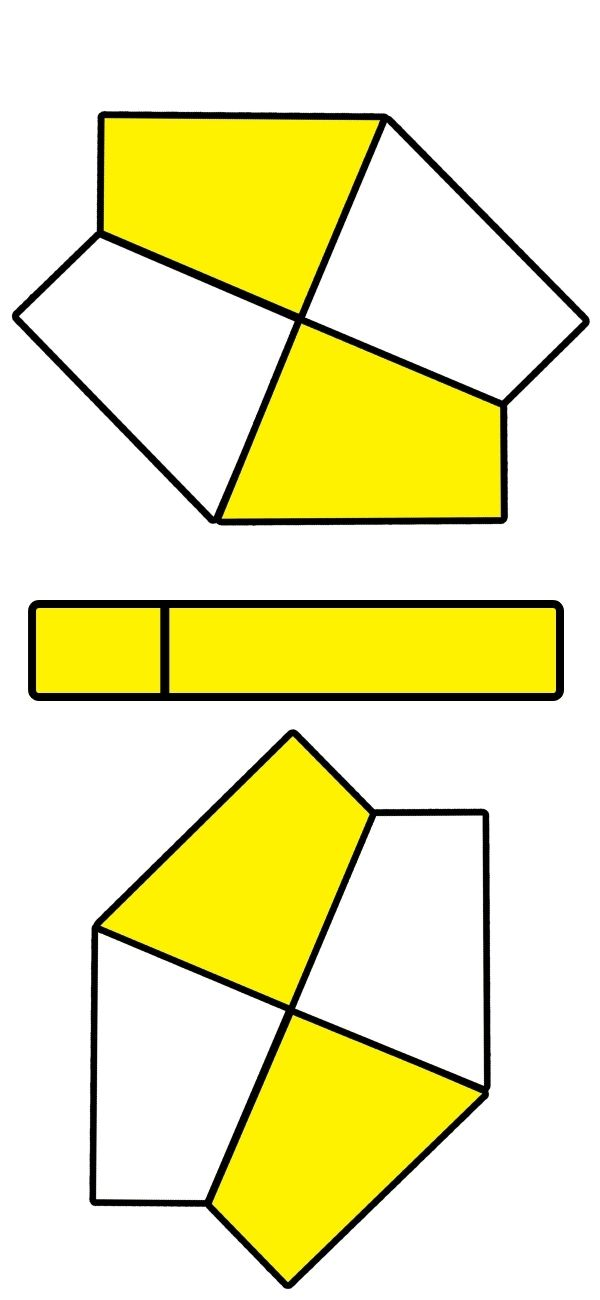### Case 4

/ (-3,-3) /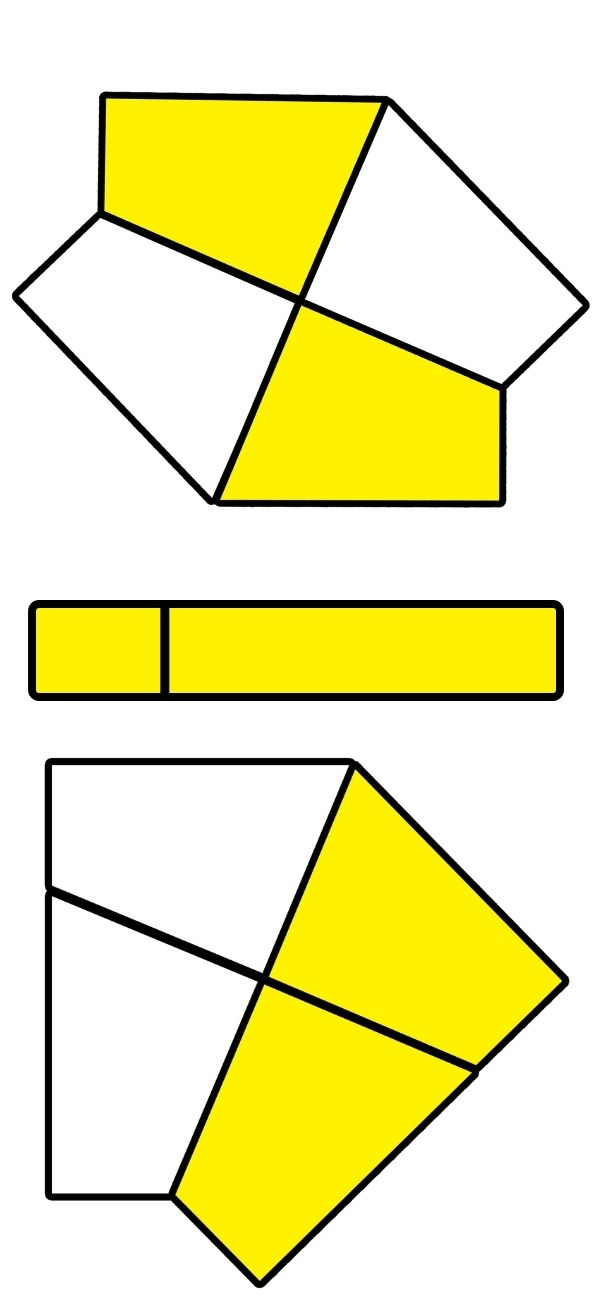### Case 5

/ (3,0) / (3,0) /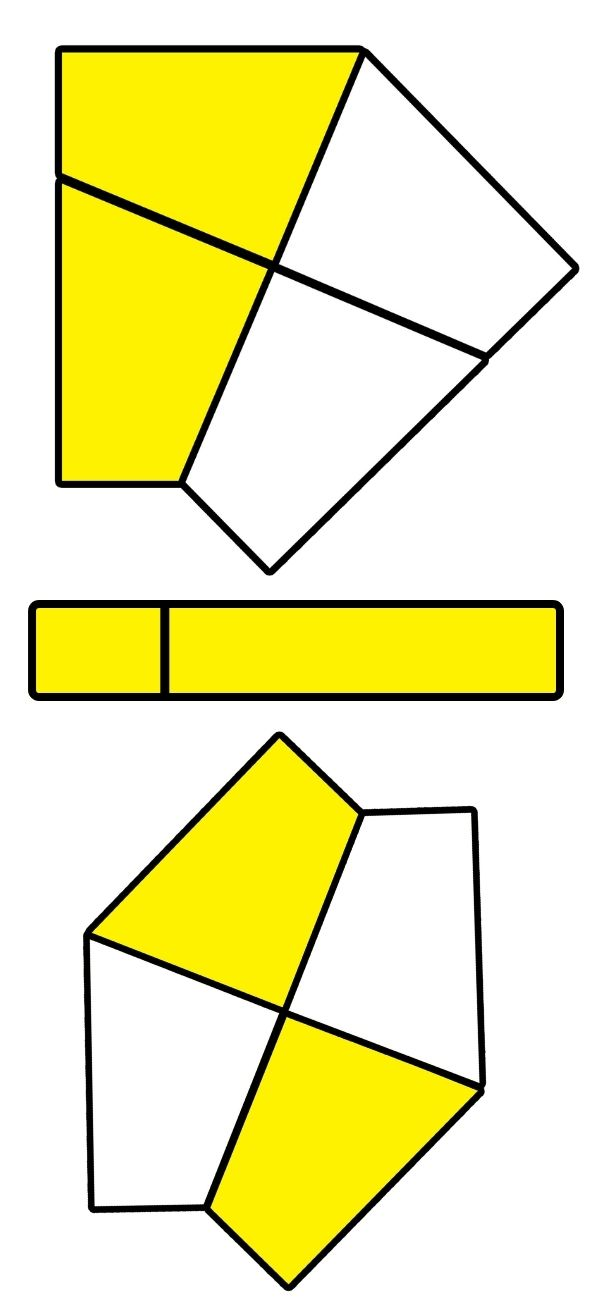### Case 6

/ (-3,0) / (0,-3) /

# Step 2

Okay, this is the big step, lets permute the corners and hopefully solve our Square-0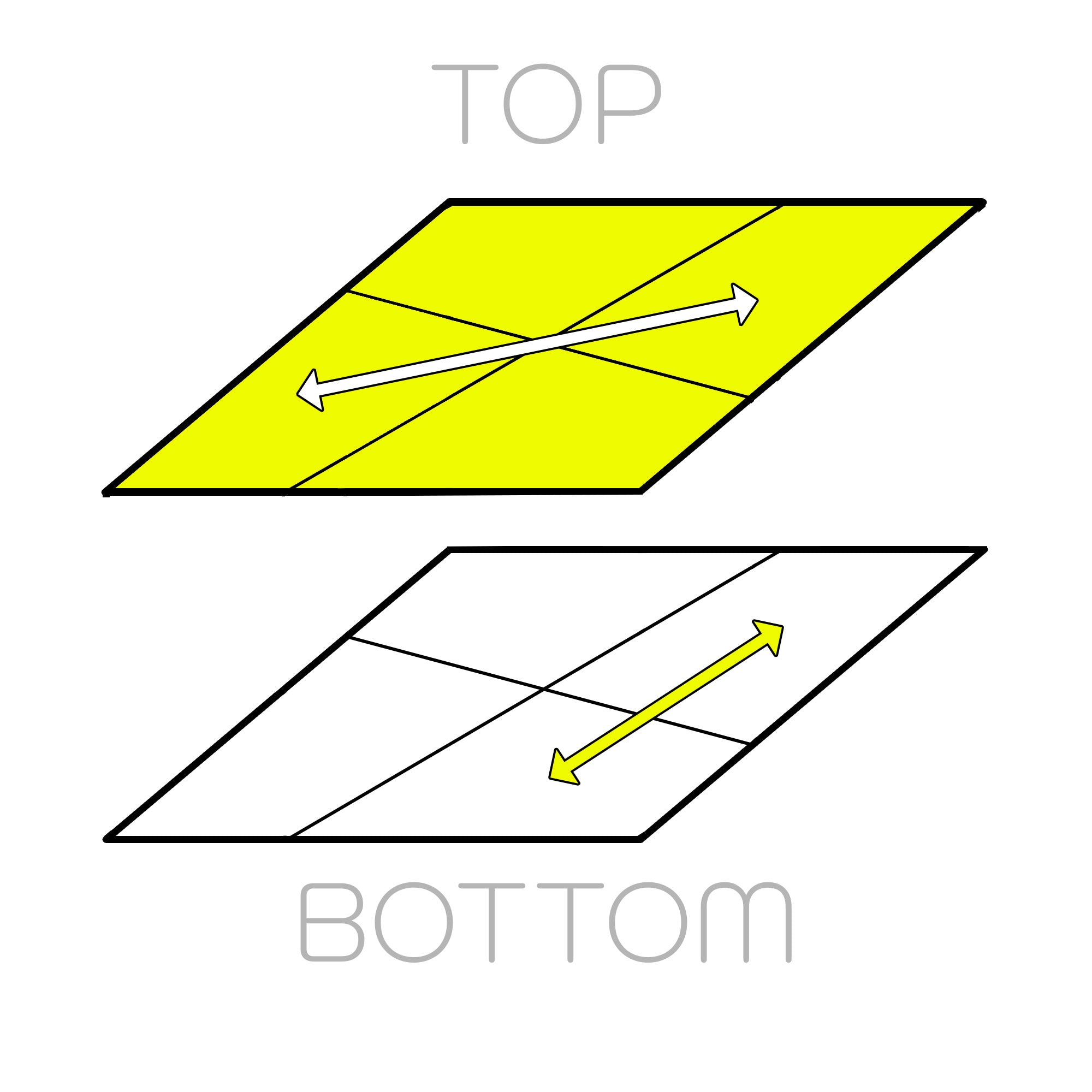### Case 1

/ (-3,0) / (3,0) / (-3,0) / (3,0) /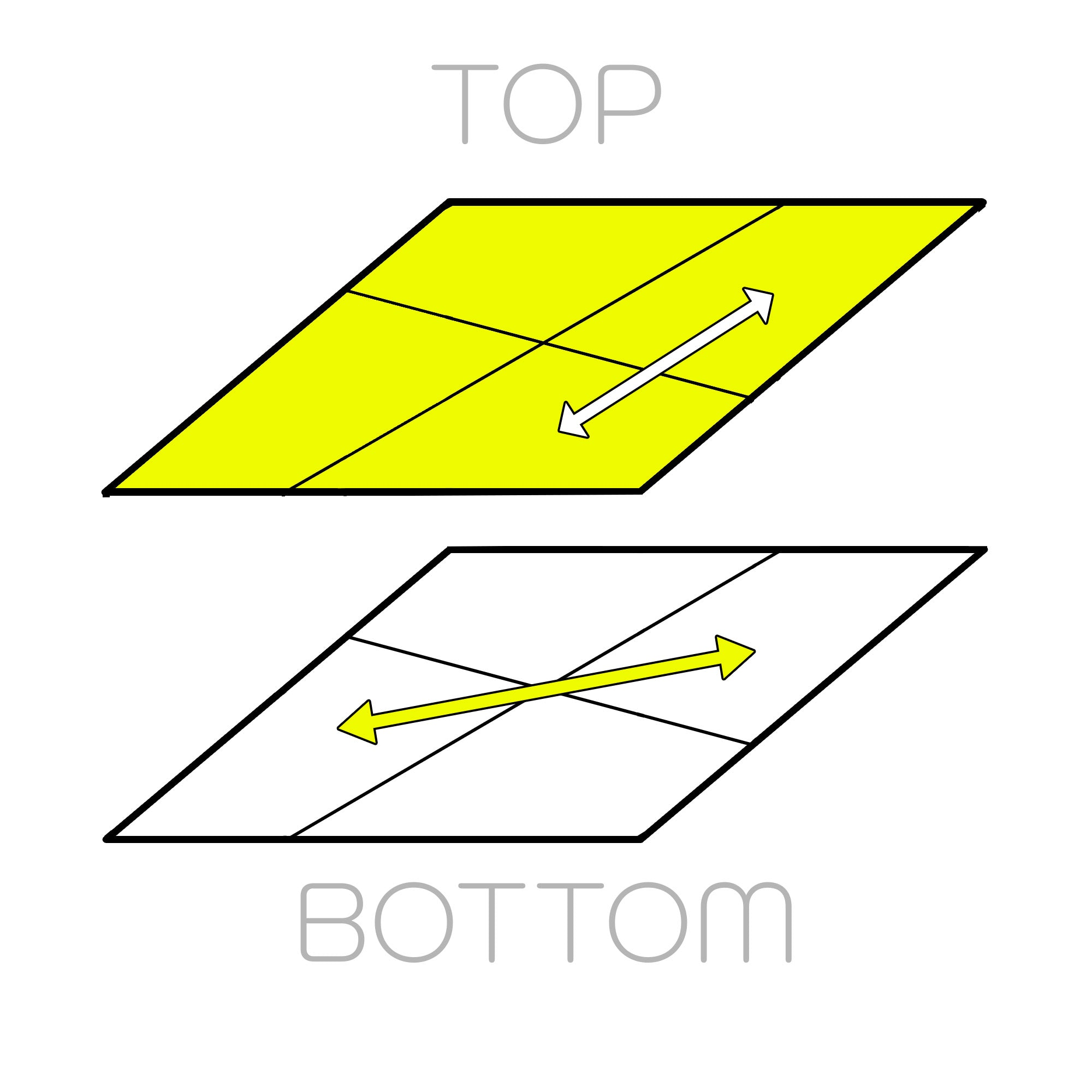### Case 2

/ (0,3) / (0,-3) / (0,3) / (0,-3) /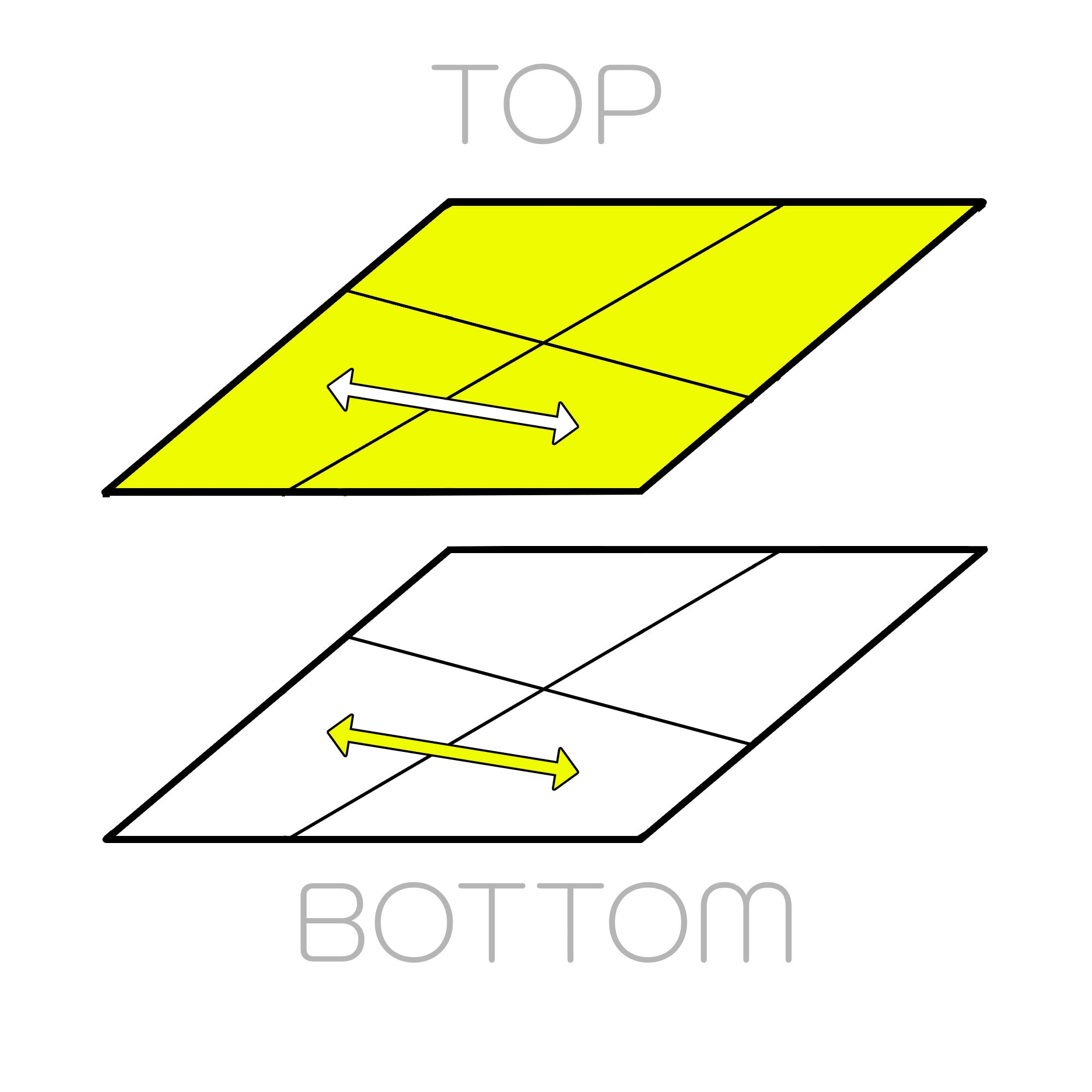### Case 3

/ (-3,0) / (3,3) / (0,-3) /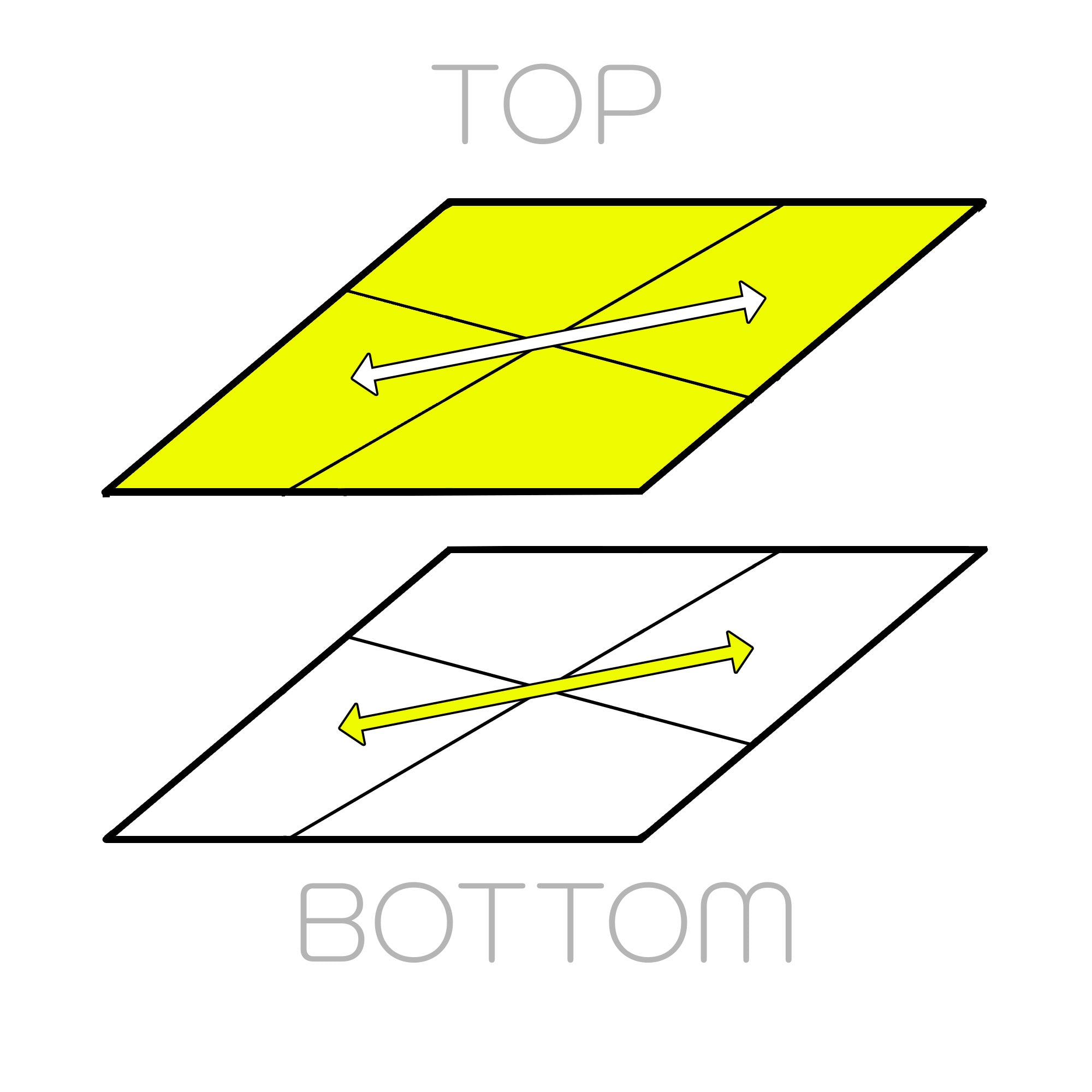### Case 4

/ (-3,3) / (3,-3) /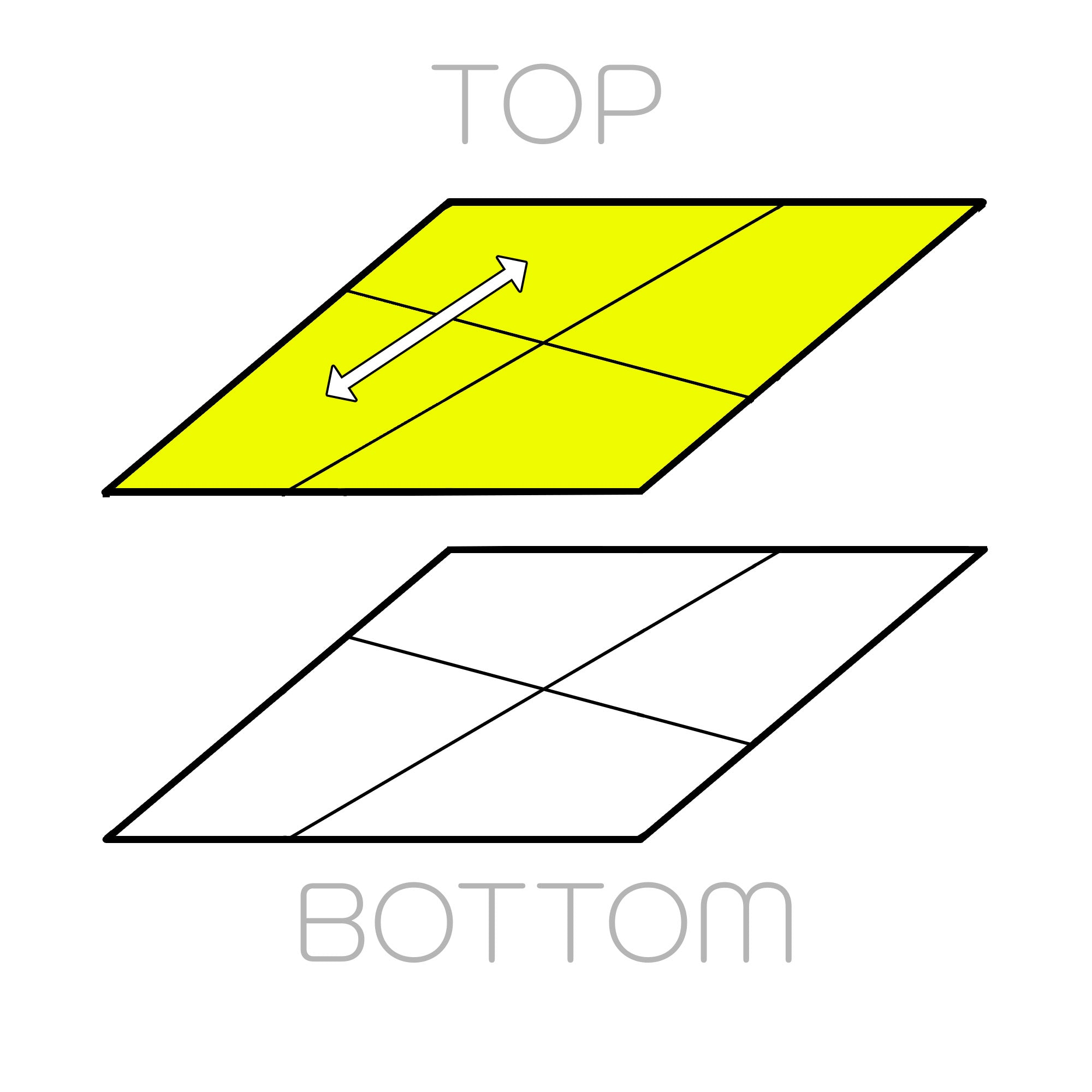### Case 5

/ (0,3) / (0,-3) / (0,3) / (-3,0) / (3,-3) /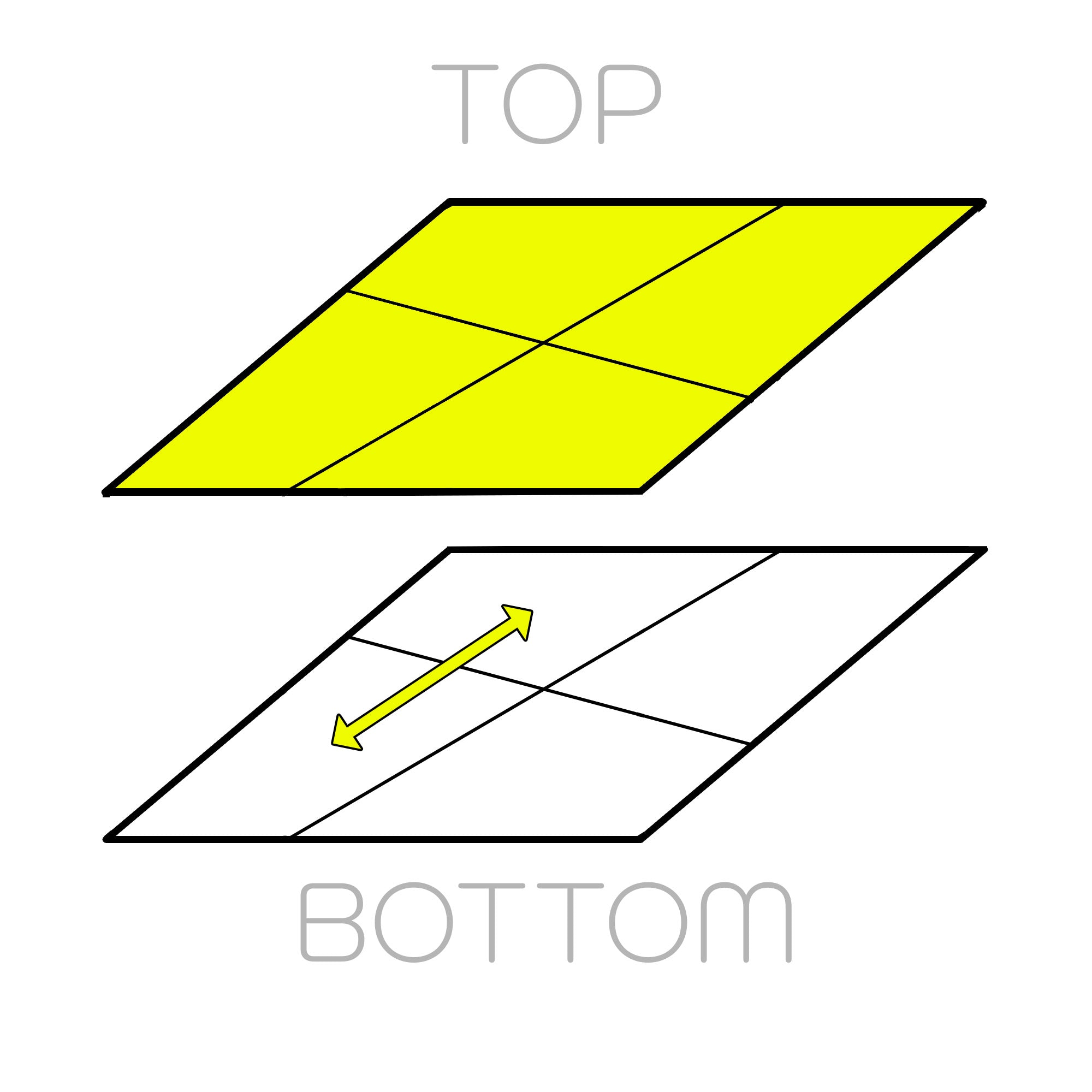### Case 6

/ (-3,0) / (3,0) / (-3,0) / (0,3) / (3,-3) /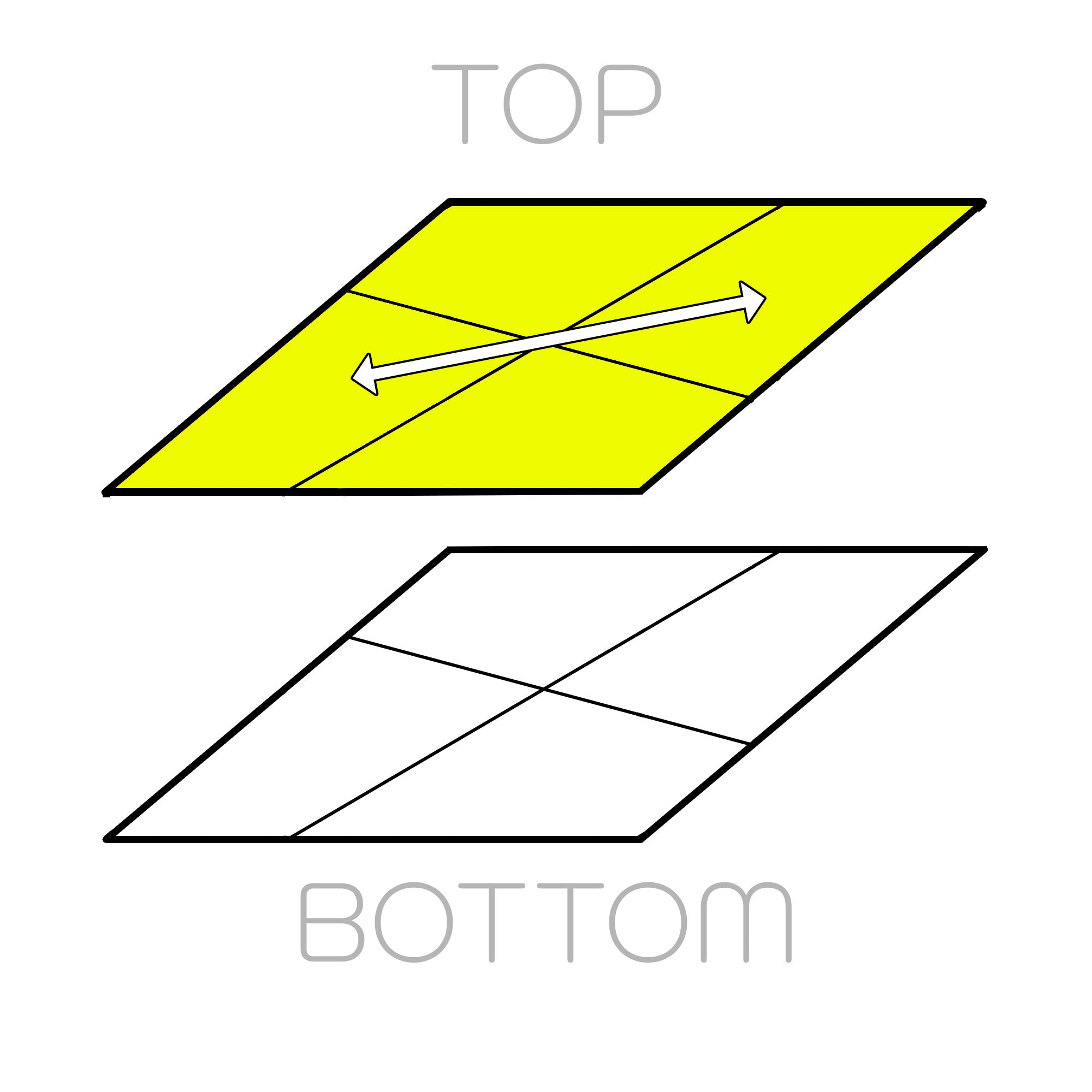### Case 7

/ (3,0) / (-3,0) / (0,3) / (0,3) / (-3,0) / (3,0) /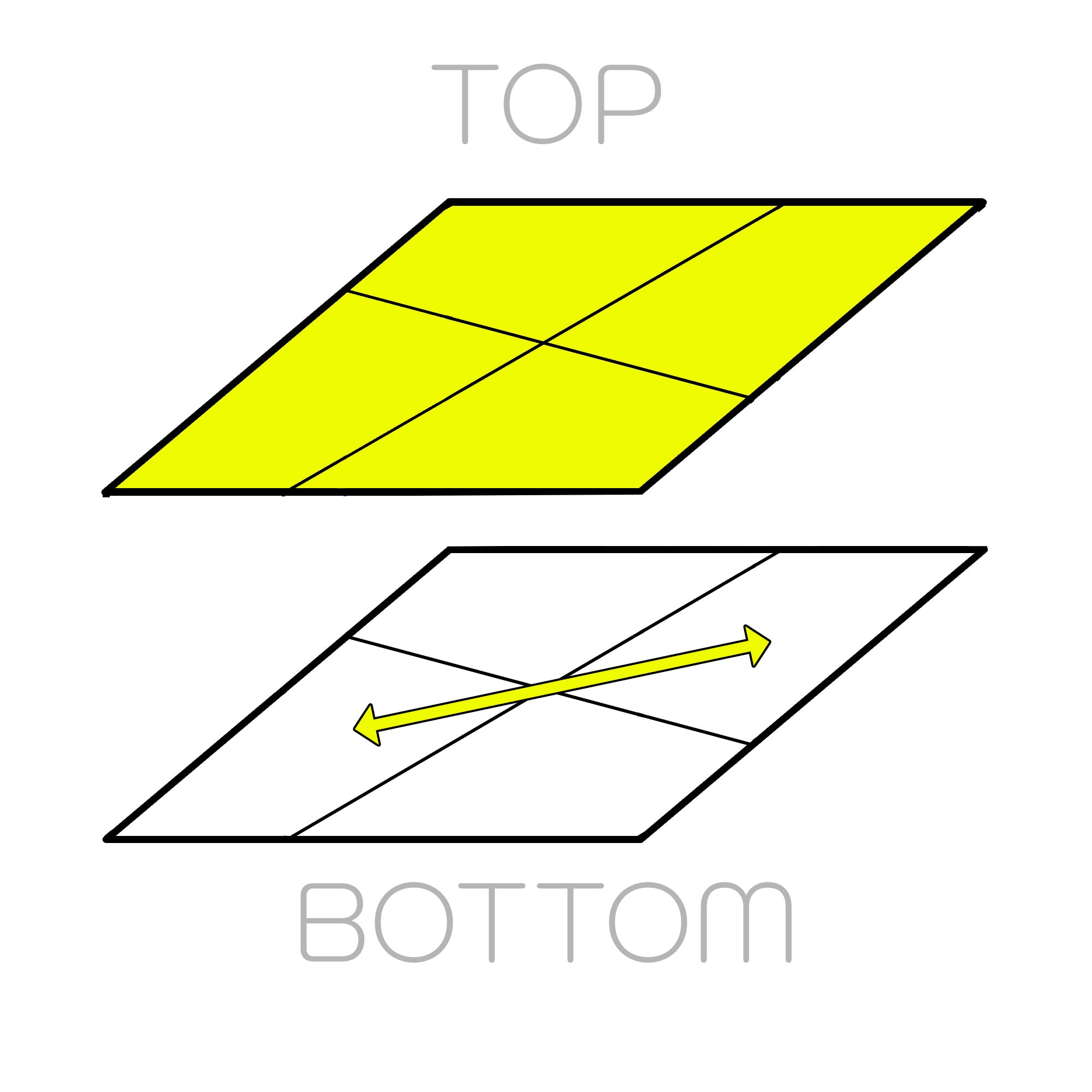### Case 8

/ (0,-3) / (0,3) / (-3,0) / (-3,0) / (0,3) / (0,-3)/

# Step 3

This is a bonus step (if you could even call it that, more like an additional step that may or may not be needed)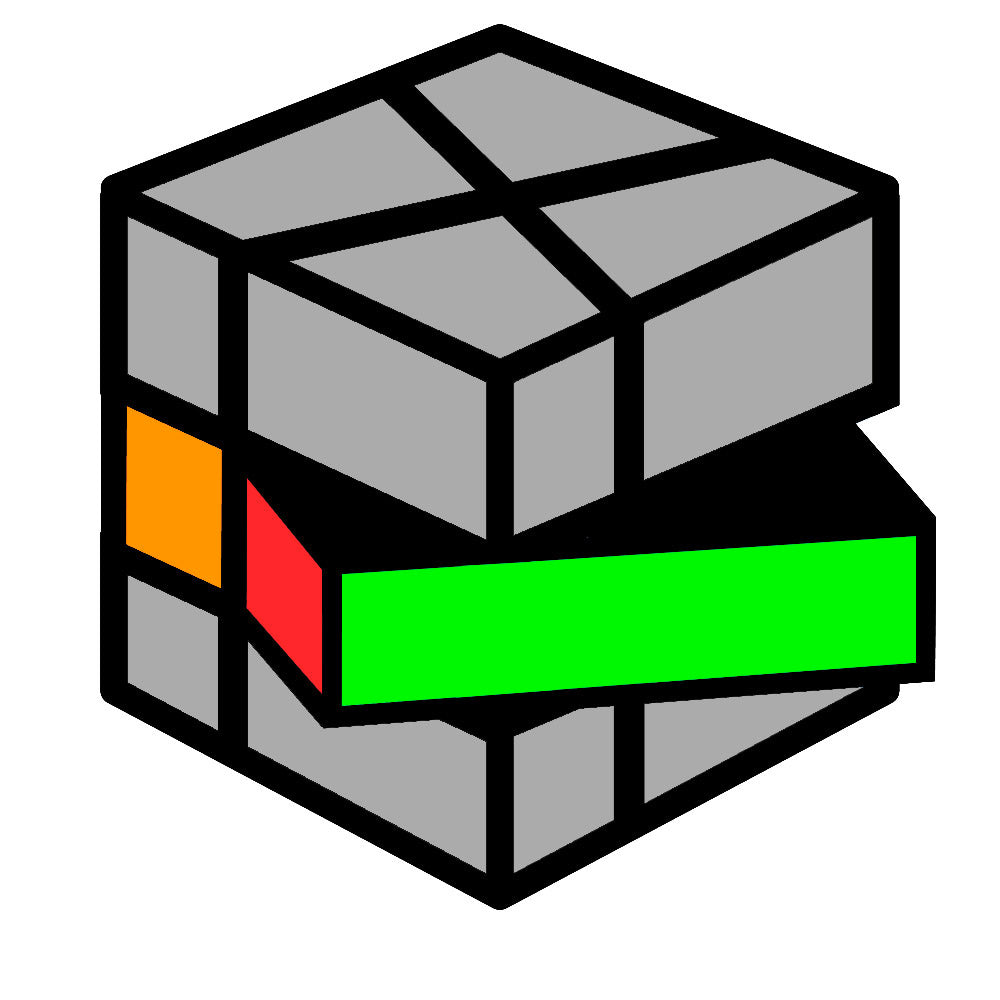### Case 1

/ (6,0) / (6,0) /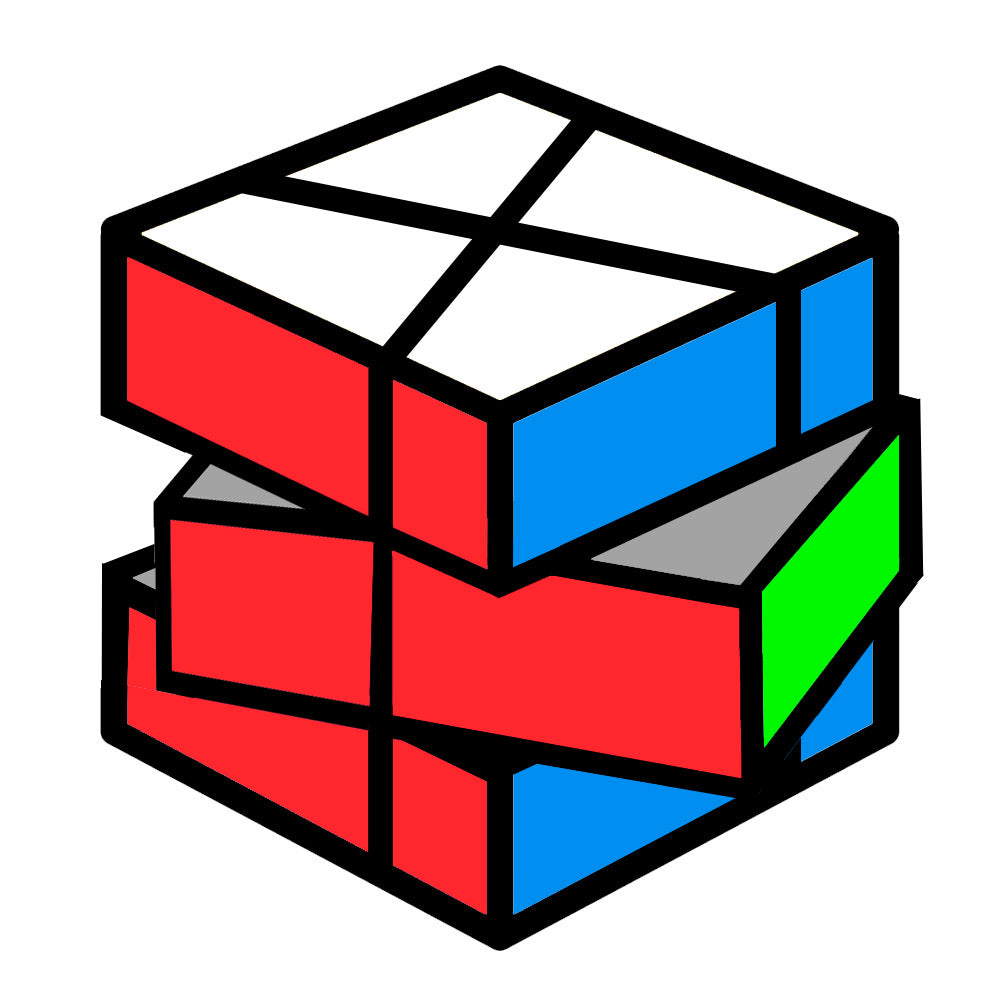/ (6,6) /